[Introduction]  [Signal arithmetic]  [Signals and noise]   [Smoothing]   [Differentiation]  [Peak Sharpening]  [Harmonic analysis]   [Fourier convolution]  [Fourier deconvolution]  [Fourier filter]  [Wavelets]   [Peak area measurement]  [Linear Least Squares]  [Multicomponent Spectroscopy]  [Iterative Curve Fitting]  [Hyperlinear quantitative absorption spectrophotometry] [Appendix and Case Studies]  [Peak Finding and Measurement]  [iPeak]   [iSignal]  [Peak Fitters]   [iFilter]  [iPower]  [List of downloadable software]  [Interactive tools]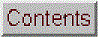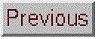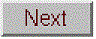## Curve fitting B: Multicomponent Spectroscopy

[Classical Least Squares (CLS) calibration]   [Inverse Least Squares (ILS) calibration]   [Spreadsheets]   [Matlab/Octave]  [Python]

The spectroscopic analysis of mixtures, when the spectra of the components overlap considerably, can be performed using special calibration methods based on a type of linear least-squares called multiple linear regression. This method is widely used in multi-wavelength instruments such as diode-array, Fourier transform, and digitally-controlled scanning spectrometers (because perfect wavelength reproducibility is a key requirement). To understand the required math, it's helpful to do a little basic matrix algebra (a.k.a., linear algebra), which is just a shorthand notation for dealing with signals expressed as equations with one term for each point. Because that area of math may not be a part of everyone's math background, I'll actually do some elementary matrix math derivations in this section, a departure from my usual orientation.

Definitions:

n = number of distinct chemical components in the mixture
s = number of samples
s1, s2 = sample 1, sample 2, etc.
c = molar concentration
c1, c2 = component 1, component 2, etc.
w = number of wavelengths at which signal is measured
w1, w2 = wavelength 1, wavelength 2, etc.
Ɛ = analytical sensitivity (slope of a plot of A vs c)
A = analytical signal
MT = matrix transpose of matrix M (rows and columns switched).
M-1 = matrix inverse of matrix M.

Assumptions:

The analytical signal, A (such as absorbance in absorption spectroscopy, fluorescence intensity in fluorescence spectroscopy, and reflectance in reflectance spectroscopy) is directly proportional to concentration, c. The proportionality constant, which is the slope of a plot of A vs c, is Ɛ.

A = Ɛc

The total signal is the sum of the signals for each component in a mixture:

Atotal = Ac1 + Ac2 + ... for all n components.

Classical Least Squares (CLS) calibration, also called the "K-matrix" method.

###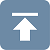This method is applicable to the quantitative analysis of a mixture of components when the spectra of the individual components are known and where total signal of the mixture is the sum of the signals for each component in the mixture. Measurement of the spectra of known concentrations of the separate components allows their analytical sensitivity Ɛ at each wavelength to be determined. Then it follows that:

Aw1=Ɛc1,w1 cc1 + Ɛc2,w1 cc2 + Ɛc3,w1 cc3 + ... for all n components.

Aw2=Ɛc1,w2 cc1 + Ɛc2,w2 cc2 + Ɛc3,w2 cc3 + ...

and so on for all wavelengths - w3, w4, etc. It's messy to write out all these individual terms, especially because there may be hundreds of wavelengths in modern array-detector spectrometers. But despite the mass of raw data, these are just really nothing more than linear equations and so the calculations required here are actually pretty simple and certainly very easy for a computer to do. So we really need a correspondingly simple notation that's more compact. To do this, we use bold-face letters to represent a vector (like a column or row of numbers in a spreadsheet) or a matrix (like a block of numbers in a spreadsheet). For example, A could represent the list of absorbances at each wavelength in a absorption spectrum. So this big set of linear equations can be written:

A = ƐC

where A is the w-length vector of measured signals (i.e. the signal spectrum) of the mixture, Ɛ is the n x w rectangular matrix of the known Ɛ-values for each of the n components at each of the w wavelengths, and C is the n-length vector of concentrations of all the components. ƐC means that Ɛ "pre-multiplies" C; that is, each column of Ɛ is multiplied point-by-point by the vector C.

If you have a sample solution containing unknown amounts of
components those n components, you measure its spectrum A and seek to calculate the concentration vector of concentrations C. In order to solve the above matrix equation for C, the number of wavelengths w must be equal to or greater than the number of components n. If w = n, then we have a system of n equations in n unknowns which can be solved by pre-multiplying both sides of the equation by Ɛ-1, the matrix inverse of Ɛ, and using the property that any matrix times its inverse is unity:
C = Ɛ-1A
Because real experimental spectra are subject to random noise (e.g. photon noise and detector noise), the solution will be more precise if the signals at a larger number of wavelengths are used, i.e. if w > n. This is easily done with no increase in labor by using a modern array-detector spectrophotometer. But then the equation can not be solved by simple matrix inversion, because the Ɛ matrix is a w x n matrix and a matrix inverse exists only for square matrices. However, a solution can be obtained in this case by pre-multiplying both sides of the equation by the expression (ƐTƐ)-1ƐT:

(ƐTƐ)-1ƐTA = (ƐTƐ)-1ƐTƐC = (ƐTƐ)-1(ƐTƐ)C

where ƐT is the transpose of Ɛ (rows and columns switched). But the quantity (ƐTƐ)-1(ƐTƐ) is a matrix times its inverse and is therefore unity. Thus:

C = (ƐTƐ)-1ƐTA

This is called the "normal equation". In this expression, ƐTƐ is a square matrix of order n, the number of components. In most practical applications, n is relatively small, typically only 2 to 5. That is not a very big matrix to invert, no matter how many wavelengths are used. In general, the more wavelengths are used, the more effectively the random noise will be averaged out (although it won't help to use wavelengths in spectral regions where none of the components produce analytical signals). The determination of the optimum wavelength region must usually be determined empirically.  All components that contribute to the spectrum must be accounted for and included in the Ɛ matrix.

Three
extensions of the CLS method are commonly made:
(a) If you have s multiple unknown samples to measure, you can compute them all at once using the same notation as above, by combining their spectra into a w x s matrix A, which will result in an n x s matrix C. (This is applied in Appendix AD: "Spectroscopy and chromatography combined: time-resolved Classical Least Squares").

(b) In order to account for flat baseline shift caused by drift, background, and light scattering, a column of 1s is added to the Ɛ matrix. This has the effect of introducing into the solution an additional component with a flat spectrum; this is referred to as "background correction".

(c) In order to account for the fact that the precision of measurement may vary with wavelength, it is common to perform a weighted least squares solution that de-emphasizes wavelength regions where precision is poor:

C = (ƐT Ɛ-1Ɛ)-1 ƐT V-1 A

where V is a w x w diagonal matrix of variances at each wavelength. In absorption spectroscopy, where the precision of measurement is poor in spectral regions where the absorbance is very high (and the light level and signal-to-noise ratio therefore low), it is common to use the transmittance T or its square T2 as weighting factors.
The method is in principle applicable to any number of overlapping components. Its accuracy is limited by how accurately the spectra of the individual components are known, the amount of noise in the signal, the extent of overlap of the spectra, and the linearity of the analytical curves of each component (the extent to which the signal amplitudes are proportional to concentration).  In practice, the method does not work well with old-style instruments with manual wavelength control, because of insufficient wavelength reproducibility. Specifically, many measurements are made on the sides of spectral bands, where even small failures in the reproducibility of wavelength settings between measurements would result in large intensity changes. But it works well with automated computer-controlled scanning instruments, and is perfectly suited to diode-array and Fourier transform instruments, which have extremely good wavelength reproducibility. The method also depends on the linearity of analytical signal with respect to concentration. The well-known deviations from analytical curve linearity in absorption spectrophotometry set a limit to the performance to this method (and which can be avoided by applying curve fitting and Fourier convolution to the transmission spectra. That idea will be developed later in this essay).

Inverse Least Squares (ILS) calibration.

###ILS is a method that can be used to measure the concentration of an analyte in samples in which the spectrum of the analyte in the sample is not known beforehand. Whereas the classical least squares method models the signal at each wavelength as the sum of the concentrations of the analyte times the analytical sensitivity, the inverse least squares methods use the reverse approach and models the analyte concentration c in each sample as the sum of the signals A at each wavelength times calibration coefficients m that express how the concentration of that component is related to the signal at each wavelength:

cs1 = mw1As1,w1 + mw2As1,w2+ mw3As1,w3 + ... for all w wavelengths.

cs2 = mw1As2,w1 + mw2As2,w2 + mw3As2,w3 + ...

and so on for all s samples. In matrix form

C = AM

where C is the s-length vector of concentrations of the analyte in the s samples, A is the w x s matrix of measured signals at the w wavelengths in the s samples, and M is the w-length vector of calibration coefficients.

Now, suppose that you have a set of standard samples that are typical of the type of sample that you wish to be able to measure and which contain a range of analyte concentrations that span the range of concentrations expected to be found in other samples of that type. This will serve as the calibration set. You measure the spectrum of each of the samples in this calibration set and put these data into a w x s matrix of measured signals A. You then measure the analyte concentrations in each of the samples by some reliable and independent analytical method and put those data into a s-length vector of concentrations C. Together these data allow you to calculate the calibration vector M by solving the above equation. If the number of samples in the calibration set is greater than the number of wavelengths, the least-squares solution is:

M = (ATA)-1ATC

(Note that ATA is a square matrix of size w, the number of wavelengths, which must be less than s). This calibration vector can be used to compute the analyte concentrations of other samples, which are similar to but not in the calibration set, from the measured spectra of the samples:

C = AM

Clearly this will work well only if the analytical samples are similar to the calibration set. The advantage of this method is that the spectrum of an unknown sample can be measured much more quickly and cheaply than the more laborious standard reference methods that are used to measure the calibration set, but as long as the unknowns are similar enough to the calibration set, the concentrations calculated by the above equation will be accurate enough for many purposes.

###Most modern spreadsheets have basic matrix manipulation capabilities and can be used for multicomponent calibration, for example Excel and OpenOffice Calc.  The spreadsheets RegressionDemo.xls and RegressionDemo.ods (for Excel and Calc, respectively) demonstrate the classical least squares procedure for a simulated spectrum of a 5-component mixture measured at 100 wavelengths. A screen shot is shown below. The matrix calculations described above that solves for the concentration of the components on the unknown mixture:
C = (ƐTƐ)-1ƐTA
are performed in these spreadsheets by the TRANSPOSE (matrix transpose), MMULT (matrix multiplication), and MINVERSE (matrix inverse) array functions, laid out step-by-step in rows 123 to 158 of this spreadsheet. Alternatively, all these array operations may be combined into one big scary cell equation:

C

= MMULT (MMULT (MINVERSE (MMULT (TRANSPOSE (
E);E)); TRANSPOSE (E));A)

where C is the vector of the 5 concentrations of all the components in the mixture, E is the 5 x 100 rectangular matrix of the known sensitivities (e.g. absorptivities) for each of the 5 components at each of the 100 wavelengths, and A is the vector of measured signals at each of the 100 wavelengths (i.e. the signal spectrum) of the unknown mixture. (Note: spreadsheet array functions like this must be entered by typing Ctrl-Shift-Enter, not just Enter as usual, See "Guidelines and examples of array formulas".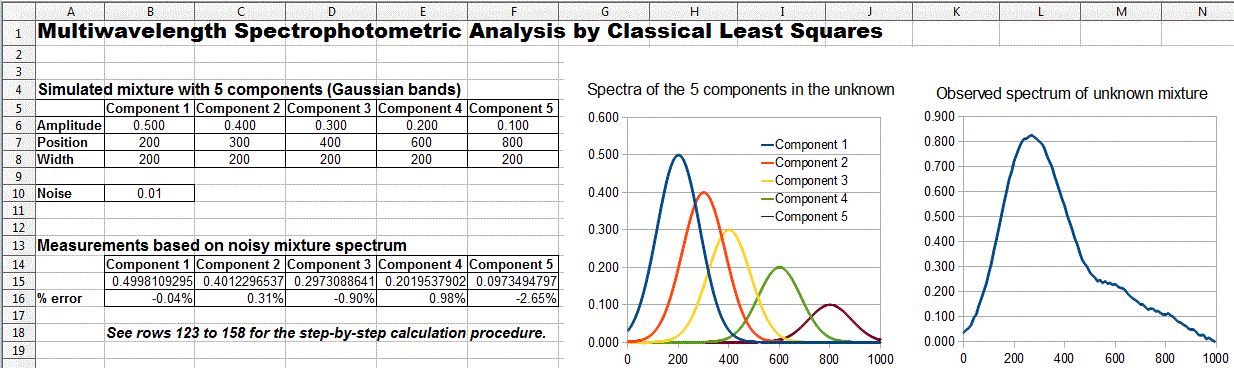.
the CLS procedure for the measurement of a 5-component unknown mixture at 100 wavelengths

Alternatively, you can skip over all the details above and use the built-in LINEST function, in both Excel or OpenOffice Calc, which performs this type of calculation in a single function statement. This is illustrated in RegressionTemplate.xls, in cell Q23. (A slight modification of the function syntax, shown in cell Q32, performs a baseline corrected calculation). A significant advantage of the LINEST function is that it can automatically compute the standard errors of the coefficients and the R2 value in the same operation; using Matlab or Octave, that would require some extra work. (LINEST is also an array function that must also be entered by typing Ctrl-Shift-Enter, not just Enter). Note that this is the same LINEST function that was previously used for polynomial least-squares; the difference is that in polynomial least-squares, the multiple columns of x values are computed, for example by taking the powers (squares, cubes, etc) of the x's, whereas in the multicomponent CLS method, the multiple columns of x values are experimental spectra of the different standard solutions. The math is the same, but the origin of the x data is very different.

A template for performing a 5-component analysis on your own data, with step-by-step instructions, in available as RegressionTemplate.xls and RegressionTemplate.ods (Graphic with example data from the demo above). Paste you own data into columns B - G. You must adjust the formulas if your number of data points or of components is different from this example. The easiest way to add more wavelengths is to select an entire row anywhere between row 40 and the end, right-click on the row number on the left and select Insert. That will insert a new blank row and will automatically adjust all the cell formulas (including the LINEST function) and the graph to include the new row.  Repeat that as many times as needed.  Finally, select the entire row just before the insertion (that is, the last non-blank row) and drag-copy in down to fill in all the new blank rows. Changing the number of components is more difficult: it involves inserting or deleting columns between C and G and between H and L, and also adjusting the formulas in rows 15 and 16 and also in Q29-U29.

Spreadsheets of this type, though easy to use once constructed, must be carefully modified for different applications that have different numbers of components or wavelengths, which is inconvenient and can be error-prone. However, it is possible to construct these spreadsheets in such a way that they automatically adjust to any number of components or wavelengths. This is done by using two new functions: (a) the COUNT function in cells B18 and F18, which counts the number of wavelengths in column A and the number of components in row Q22-U22, respectively, and (b) the INDIRECT function in cell Q23 and in row 12 and 13, which allows the address of a cell or range of cells to be calculated within the spreadsheet (based on the number of wavelengths and components just counted) rather than using a fixed address range. This technique is used in RegressionTemplate2.xls and in two examples showing the same template with data entered for different numbers of wavelengths and for mixtures of 5 components at 100 wavelengths (RegressionTemplate2Example.xls) and for 2 components at 59 wavelengths (RegressionTemplate3Example.xls). You'll still have to adjust the graph, however, to cover the desired x-axis range.

Excel template applied to the measurement of a 5-component unknown mixture at 100 wavelengths.

###Matlab or Octave

Matlab and Octave are really the natural computer approaches to multicomponent analysis because they handle all types of matrix math so easily, compactly, and quickly. In these languages, the notation is very compact: the transpose of matrix A is A', the inverse of A is inv(A), and matrix multiplication is designated by an asterisk (*). Thus the solution to the classical least squares method above is written in Matlab/Octave notation as

C = inv(E'*E)*E'*A

where E is the rectangular matrix of sensitivities at each wavelength for each component and A is the observed spectrum of the mixture. Note that the Matlab/Octave notation is not only shorter than the spreadsheet notation, it's also closer to the traditional mathematical notation. Even more compactly, you can write C = A/E, using the Matlab forward slash or "right divide" operator, which yields the same results but is in principle more accurate with respect to the numerical precision of the computer (usually negligible compared to the noise in the signal; see CaseStudies.html#Numerical).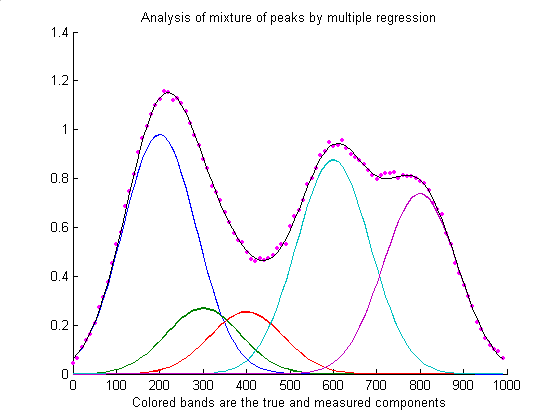The script RegressionDemo.m (for Matlab or Octave) demonstrates the classical least squares procedure for a simulated absorption spectrum of a 5-component mixture at 100 wavelengths, illustrated above. Most of this script is just signal generation and plotting; the actual least-squares regression is performed on one line:
`MeasuredAmp = ObservedSpectrum*A'*inv(A*A')`
where different symbols are used for the variables: "A" is a matrix containing the spectrum of each of the components in each of its rows and "ObservedSprectum" is the observed spectrum of the unknown mixture. In this example the dots represent the observed spectrum of the mixture (with noise) and the five colored bands represent the five components in the mixture, whose spectra are known but whose concentrations in the mixture are unknown. The black line represents the "best fit" to the observed spectrum calculated by the program. In this example the concentrations of the five components are measured to an accuracy of about 1% relative (limited by the noise in the observed spectrum).  Comparing RegressionDemo.m to its spreadsheet equivalent, RegressionDemo.ods, both running the same  computer, you can see that the Matlab/Octave code computes and plots the results quicker than the spreadsheet, although both take no more than a fraction of a second for this example.

Extensions:
(a) The extension to multiple unknown samples, each with its own "ObservedSpectrum" is straightforward in Matlab/Octave. If you have "s" samples, just assemble their observed spectra onto a matrix with "s" rows and "w" columns ("w" is the number of wavelengths), then use the same formulation as before:
`MeasuredAmp = ObservedSpectrum*A'*inv(A*A')`
and the resulting "MeasuredAmp" will be an "s" x "n" matrix rather than an n-length vector ("n" is the number of measured components). This is a great example of the convenience of the vector/matrix nature of this language.  (RegressionDemoMultipleSamples.m demonstrates this, as well as Appendix AD: "Spectroscopy and chromatography combined: time-resolved Classical Least Squares").

(b) The extension to "background correction" is easily accomplished in Matlab/Octave by adding a column of 1s to the A matrix containing the absorption spectrum of each of the components.:

background=ones(size(
ObservedSpectrum));

A=[background A1 A2 A3];

where A1, A2, A3... are the absorption spectra of the individual components.

(c) Performing a T-weighted regression is also readily performed:

MeasuredAmp=([T T] .* A)\(ObservedSpectrum .* T);

where T is the transmission spectrum.  Here, the matrix division backslash "\" is used as a short-cut to the classical least-squares matrix solution (c.f. http://www.mathworks.com/help/techdoc/ref/mldivide.html).

###The cls.m function: Ordinarily, the calibration matrix M is assembled from the experimentally measured signals (e.g. spectra) of the individual components of the mixture, but it is also possible to fit a computer-generated model of basic peak shapes (e.g. Gaussians, Lorentzians, etc) to a signal to determine if that signal can be represented as the weighted sum of overlapping basic peak shapes. The function cls.m computes such a model consisting of the sum of any number of peaks of known shape, width, and position, but of unknown height, and fit it to noisy x,y data sets. The syntax is

heights=cls(x, y, NumPeaks, PeakShape, Positions, Widths, extra)

where x and y are the vectors of measured data (e.g. x might be wavelength and y might be the absorbance at each wavelength), 'NumPeaks' is the number of peaks, 'PeakShape' is the peak shape number (1=Gaussian, 2=Lorentzian, 3=logistic, 4=Pearson, 5=
exponentially broadened Gaussian; 6=equal-width Gaussians; 7=Equal-width Lorentzians; 8=exponentially broadened equal-width Gaussian, 9=exponential pulse, 10=sigmoid, 11=Fixed-width Gaussian, 12=Fixed-width Lorentzian; 13=Gaussian/Lorentzian blend; 14=BiGaussian, 15=BiLorentzian), 'Positions' is the vector of peak positions on the x axis (one entry per peak), 'Widths' is the vector of peak widths in x units (one entry per peak), and 'extra' is the additional shape parameter required by the exponentially broadened, Pearson, Gaussian/Lorentzian blend, BiGaussain and BiLorentzian shapes. Cls.m returns a vector of measured peak heights for each peak.

The
demonstration script clsdemo.m (Graphic) creates some noisy model data, fits it with cls.m, computes the accuracy of the measured heights, then repeats the calculation by iterative non-linear least squares peak fitting (INLS, covered in the next section) using the downloadable Matlab/Octave function peakfit.m, making use of the known peak positions and widths only as starting guesses ("start"). You can see that CLS is faster and (usually) more accurate, especially if the peaks are highly overlapped. (This script requires cls.m, modelpeaks.m, and peakfit.m in the Matlab/Octave path).
Figure(1): Classical Least Squares (multilinear regression)
Elapsed time is 0.000937 seconds.
Average peak height accuracy = 0.9145%

Figure(2): Iterative non-linear peak fitting with peakfit.m
Elapsed time is 0.171765 seconds.
Average peak height accuracy = 1.6215%
On the other hand, INLS can be better than CLS if there are unsuspected shifts in peak position and/or peak width between calibration and measurement (for example caused by drifting spectrometer calibration or by changing temperature, pressure, or solution variables) because INLS can track and compensate for changes in peak position and width. You can test this by changing the variable "PeakShift" (line 16) to a non-zero value in clsdemo.m.

The cls2.m function is similar to cls.m, except that it also measures the baseline (assumed to be flat), using
extension to "background correction" described above, and returns a vector containing the background B and measured peak heights H for each peak , e.g. [B H1 H2 H3...].

Weighted linear regression:
The downloadable Matlab/Octave function "tfit.m" simulates the measurement of the absorption spectrum of a mixture of three components by weighted linear regression (on line 61), demonstrates the effect of  the amount of noise in the signal, the extent of overlap of the spectra, and the linearity of the analytical curves of each component. This function also compares the results to a more advanced method described later (line 66) that applies curve fitting to the transmission spectra rather than to the absorbance spectra.

### Classical Least Squares in Python

The equivalent expression in Python for the "Normal Equation" is

C = inv(E.T.dot(E)).dot(E.T).dot(A)

where "inv()" means the matrix inverse, "E.T"means the transpose of matrix E, and the expression ".dot" means the dot product. This is not so different than the Matlab version.

C = inv(E'* E)*E'*A

Just remember that before doing this in Python you must do the following imports:

numpy as np

from numpy.linalg import inv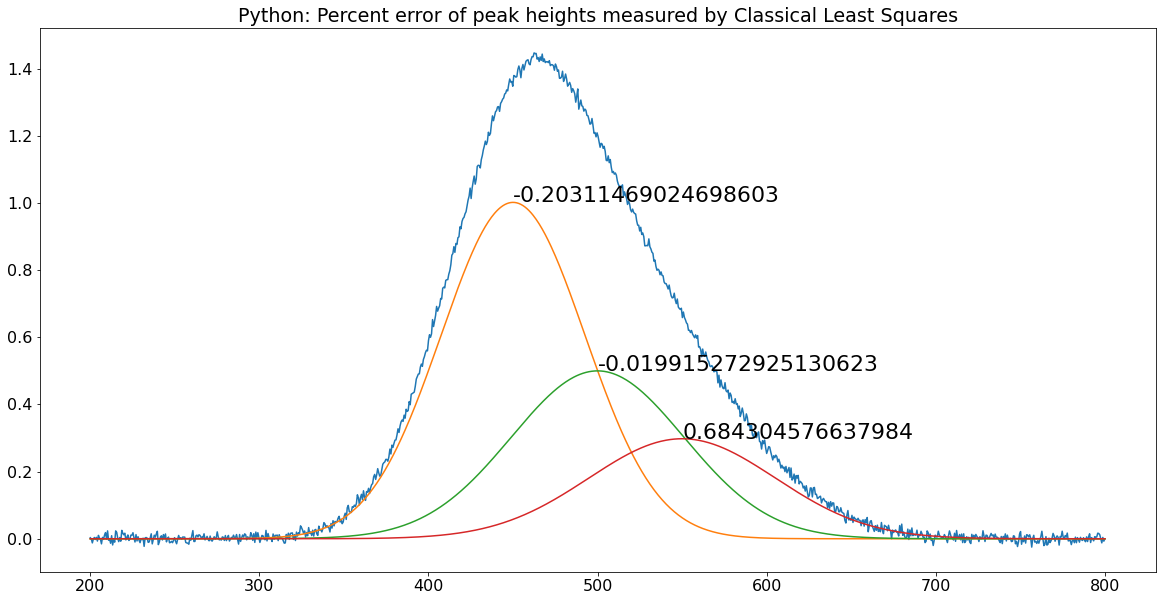The pair of matched scripts, NormalEquationDemo.py and NormalEquationDemo.m compare these aspects in Python and in Matlab. Both scripts use the same sequence of operations and the same variable names to create and plot a noisy simulated signal vector A that is the sum of three peaks of known shape but unknown amplitude, and then to measure the amplitudes C in the noisy signal by Classical Least Squares. The percent error between the true and measured peak heights are printed next to each peak. The code similarities are striking and the execution times are the same.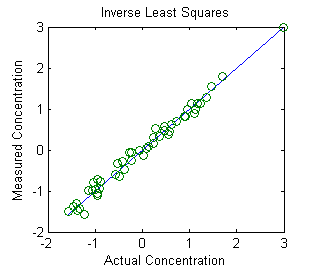###The Inverse Least Squares (ILS) technique is demonstrated in Matlab by this script and the graph above. The math, described above, is similar to the Classical Least Squares method, and can be done by any of the Matlab/Octave or spreadsheet methods described in this section. This example is a real data set derived from the near infrared (NIR) reflectance spectroscopy of agricultural wheat samples analyzed for protein content. In this example there are 50 calibration samples measured at 6 wavelengths. These calibration samples had already been analyzed for protein content by a reliable, but laborious and time-consuming, reference method.  The purpose of this calibration is to establish whether near-infrared reflectance spectroscopy, which can be measured on wheat paste preparations much more quickly than wet chemical methods, correlates to their protein content as determined by the reference method. These results indicate that it does, at least for this set of 50 wheat samples, and therefore is it likely that near-infrared spectroscopy should do a pretty good job of estimating the protein content of similar unknown samples. The key is that the unknown samples must be similar to the calibration samples (except for the protein content), but this is a very common analytical situation in industrial and agricultural quality control, where large numbers of samples of products of a similar predictable type must often be tested quickly and cheaply.